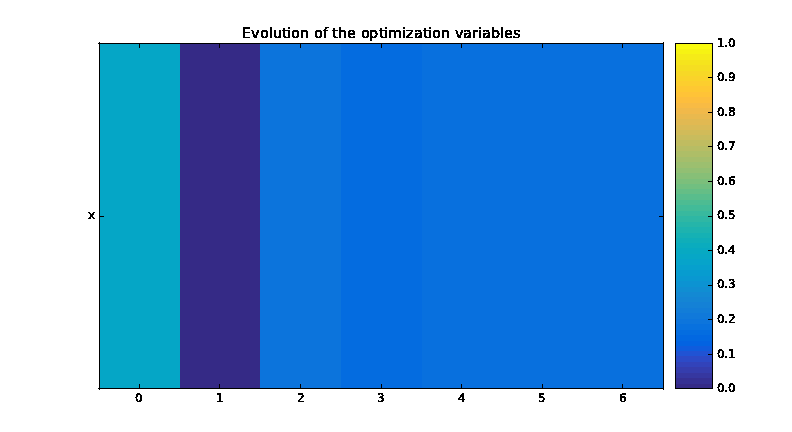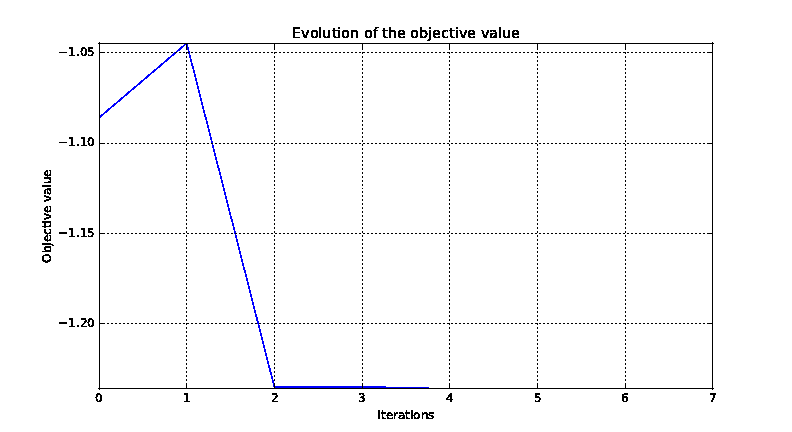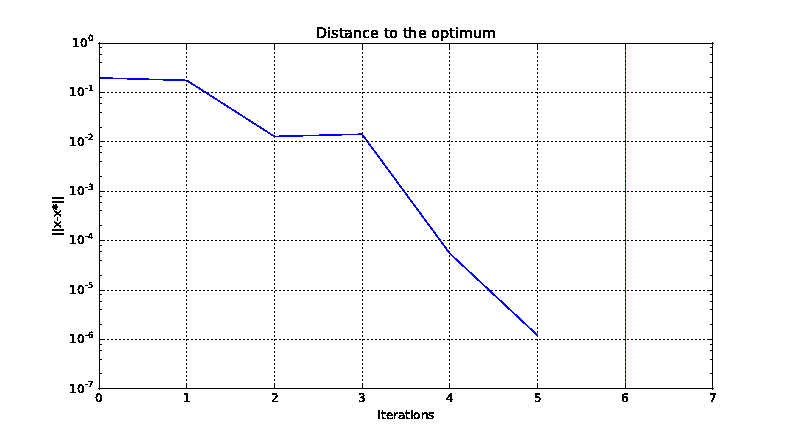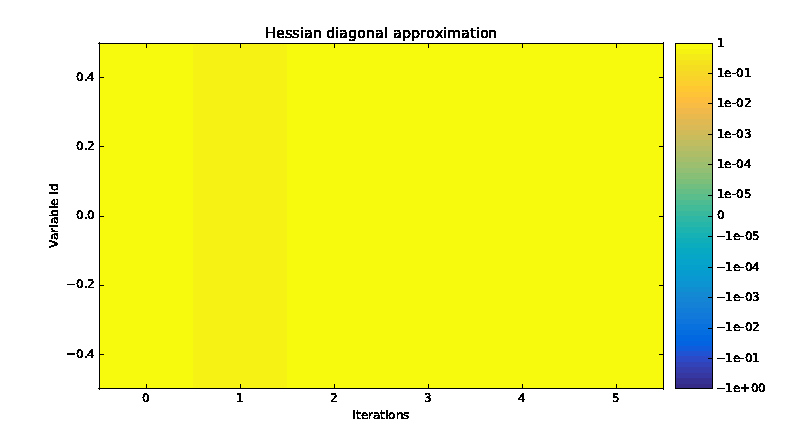# Tutorial: How to solve an optimization problem¶

Although the library |g| is dedicated to the MDO, it can also be used for mono-disciplinary optimization problems. This tutorial presents some examples on analytical test cases.

## 1. Optimization based on a design of experiments¶

Let $$(P)$$ be a simple optimization problem:

\begin{split}(P) = \left\{ \begin{aligned} & \underset{x\in\mathbb{N}^2}{\text{minimize}} & & f(x) = x_1 + x_2 \\ & \text{subject to} & & -5 \leq x \leq 5 \end{aligned} \right.\end{split}

In this subsection, we will see how to use |g| to solve this problem $$(P)$$ by means of a Design Of Experiments (DOE)

### 1.a. Define the objective function¶

Firstly, by means of the create_discipline() API function, we create a MDODiscipline of AutoPyDiscipline type from a python function:

from gemseo.api import create_discipline

def f(x1=0., x2=0.):
y = x1 + x2
return y

discipline = create_discipline("AutoPyDiscipline", py_func=f)


Now, we want to minimize this MDODiscipline over a design of experiments (DOE).

### 1.b. Define the design space¶

For that, by means of the create_design_space() API function, we define the DesignSpace $$[-5, 5]\times[-5, 5]$$ by using its add_variable() method.

from gemseo.api import create_design_space

design_space = create_design_space()
design_space.add_variable("x1", 1, l_b=-5, u_b=5, var_type="integer")
design_space.add_variable("x2", 1, l_b=-5, u_b=5, var_type="integer")


### 1.c. Define the DOE scenario¶

Then, by means of the create_scenario() API function, we define a DOEScenario from the MDODiscipline and the DesignSpace defined above:

from gemseo.api import create_scenario

scenario = create_scenario(
discipline, "DisciplinaryOpt", "y", design_space, scenario_type="DOE"
)


### 1.d. Execute the DOE scenario¶

Lastly, we solve the OptimizationProblem included in the DOEScenario defined above by minimizing the objective function over a design of experiments included in the DesignSpace. Precisely, we choose a full factorial design of size $$11^2$$:

scenario.execute({"algo": "fullfact", "n_samples": 11**2})


The optimum results can be found in the execution log. It is also possible to extract them by invoking the get_optimum() method. It returns a dictionary containing the optimum results for the scenario under consideration:

opt_results = scenario.get_optimum()
print("The solution of P is (x*,f(x*)) = ({}, {})".format(
opt_results.x_opt, opt_results.f_opt
))


which yields:

The solution of P is (x*,f(x*)) = ([-5, -5], -10.0).


## 2. Optimization based on a quasi-Newton method by means of the library scipy¶

Let $$(P)$$ be a simple optimization problem:

\begin{split}(P) = \left\{ \begin{aligned} & \underset{x}{\text{minimize}} & & f(x) = \sin(x) - \exp(x) \\ & \text{subject to} & & -2 \leq x \leq 2 \end{aligned} \right.\end{split}

In this subsection, we will see how to use |g| to solve this problem $$(P)$$ by means of an optimizer directly used from the library scipy.

### 2.a. Define the objective function¶

Firstly, we create the objective function and its gradient as standard python functions:

import numpy as np
from gemseo.api import create_discipline

def g(x=0):
y = np.sin(x) - np.exp(x)
return y

def dgdx(x=0):
y = np.cos(x) - np.exp(x)
return y


### 2.b. Minimize the objective function¶

Now, we can to minimize this MDODiscipline over its design space by means of the L-BFGS-B algorithm implemented in the function scipy.optimize.fmin_l_bfgs_b.

from scipy import optimize

x_0 = -0.5 * np.ones(1)
opt = optimize.fmin_l_bfgs_b(g, x_0, fprime=dgdx, bounds=[(-.2, 2.)])
x_opt, f_opt, _ = opt


Then, we can display the solution of our optimization problem with the following code:

print("The solution of P is (x*,f(x*)) = ({}, {})".format(x_opt, f_opt))


which gives:

The solution of P is (x*,f(x*)) = (-0.2, -1.01740008).


You can found the scipy implementation of the L-BFGS-B algorithm by clicking here.

## 3. Optimization based on a quasi-Newton method by means of the GEMSEO optimization interface¶

Let $$(P)$$ be a simple optimization problem:

\begin{split}(P) = \left\{ \begin{aligned} & \underset{x}{\text{minimize}} & & f(x) = \sin(x) - \exp(x) \\ & \text{subject to} & & -2 \leq x \leq 2 \end{aligned} \right.\end{split}

In this subsection, we will see how to use |g| to solve this problem $$(P)$$ by means of an optimizer from scipy called through the optimization interface of |g|.

### 3.a. Define the objective function¶

Firstly, by means of the create_discipline() API function, we create a MDODiscipline of AutoPyDiscipline type from a python function:

import numpy as np
from gemseo.api import create_discipline

def g(x=0):
y = np.sin(x) - np.exp(x)
return y

def dgdx(x=0):
y = np.cos(x) - np.exp(x)
return y

discipline = create_discipline("AutoPyDiscipline", py_func=g, py_jac=dgdx)


Now, we can to minimize this MDODiscipline over a design space, by means of a quasi-Newton method from the initial point $$0.5$$.

### 3.b. Define the design space¶

For that, by means of the create_design_space() API function, we define the DesignSpace $$[-2., 2.]$$ with initial value $$0.5$$ by using its add_variable() method.

from gemseo.api import create_design_space

design_space = create_design_space()
design_space.add_variable("x", 1, l_b=-2., u_b=2., value=-0.5 * np.ones(1))


### 3.c. Define the optimization problem¶

Then, by means of the create_scenario() API function, we define a MDOScenario from the MDODiscipline and the DesignSpace defined above:

from gemseo.api import create_scenario

scenario = create_scenario(
discipline, "DisciplinaryOpt", "y", design_space, scenario_type="MDO"
)


### 3.d. Execute the optimization problem¶

Lastly, we solve the OptimizationProblem included in the MDOScenario defined above by minimizing the objective function over the DesignSpace. Precisely, we choose the L-BFGS-B algorithm implemented in the function scipy.optimize.fmin_l_bfgs_b and indirectly called by means of the class OptimizersFactory and of its function execute():

scenario.execute({"algo": "L-BFGS-B", "max_iter": 100})


The optimization results are displayed in the log file. They can also be obtained using the following code:

opt_results = scenario.get_optimum()
print("The solution of P is (x*,f(x*)) = ({}, {})".format(
opt_results.x_opt, opt_results.f_opt
))


which yields:

The solution of P is (x*,f(x*)) = (-1.29, -1.24).


You can found the scipy implementation of the L-BFGS-B algorithm algorithm by clicking here.

Tip

In order to get the list of available optimization algorithms, use:

from gemseo.api import get_available_opt_algorithms

algo_list = get_available_opt_algorithms()
print('Available algorithms: {}'.format(algo_list))


what gives:

Available algorithms: ['NLOPT_SLSQP', 'L-BFGS-B', 'SLSQP', 'NLOPT_COBYLA', 'NLOPT_BFGS', 'NLOPT_NEWUOA', 'TNC', 'P-L-BFGS-B', 'NLOPT_MMA', 'NLOPT_BOBYQA', 'ODD']


## 4. Saving and post-processing¶

After the resolution of the OptimizationProblem, we can export the results into a HDF file:

problem = scenario.formulation.opt_problem
problem.export_hdf("my_optim.hdf5")


We can also post-process the optimization history by means of the function execute_post(), either from the OptimizationProblem:

from gemseo.api import execute_post

execute_post(problem, "OptHistoryView", save=True, file_path="opt_view_with_doe")


or from the HDF file created above:

from gemseo.api import execute_post

execute_post("my_optim.hdf5", "OptHistoryView", save=True, file_path="opt_view_from_disk")


This command produces a series of PDF files: